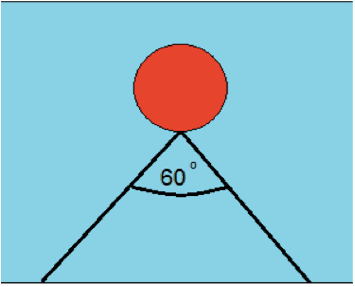# Working out tension of string holding a ball underwater

• Alice Martin

#### Alice Martin

I have a physics homework question that i need help with;
A submerged ball of negligible mass is attached to the bottom of a swimming pool by two strings as shown. The volume of the ball is 5000cm^3. Calculate the tension in each string. (hint you need to look up the density of water)

How do i do this? Do i use the buoyancy of the ball or the tension formula?

Thetriangle with the base being the bottom of the pool and the ball being attached to the top vertex with a 60 degree angle

What you need to do is a force balance on the ball.So there is a gravitational force, a buoyancy force and tension forces all acting on the ball, which is not moving. What does that tell you?

•Alice Martin
What you need to do is a force balance on the ball.So there is a gravitational force, a buoyancy force and tension forces all acting on the ball, which is not moving. What does that tell you?
Thank you! That is a really simple way of putting it. I did not even think about the fact that the forces must be equal!Hi Alice! Welcome to PF!

I've gone ahead moved your thread to the homework forums, but in the future please make sure you place your question here (or in whichever homework forum is appropriate) and that you fill in the template provided when you create a thread in the homework forums.

@mfig, please use the report feature to report homework posts outside of the homework forums instead of replying to them. Thank you!